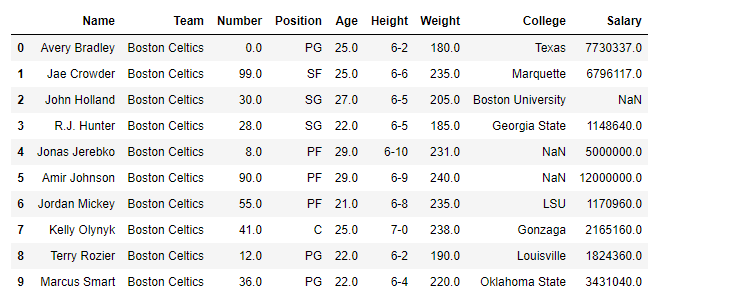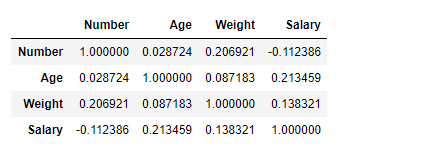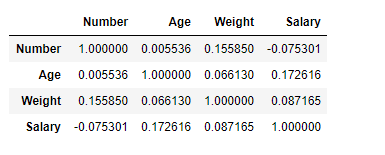Open in App
Not now

# Python | Pandas dataframe.corr()

• Difficulty Level : Easy
• Last Updated : 08 Aug, 2022

Pandas dataframe.corr() is used to find the pairwise correlation of all columns in the Pandas Dataframe in Python. Any NaN values are automatically excluded. Any non-numeric data type or columns in the Dataframe, it is ignored.

## Syntax of dataframe.corr()

Use corr() function to find the correlation among the columns in the Dataframe using the ‘Pearson’ method.

Syntax: DataFrame.corr(self, method=’pearson’, min_periods=1)

Parameters:

• method :
• pearson: standard correlation coefficient
• kendall: Kendall Tau correlation coefficient
• spearman: Spearman rank correlation
• min_periods : Minimum number of observations required per pair of columns to have a valid result. Currently only available for pearson and spearman correlation

Returns: count :y : DataFrame

### Example

A simple example to show how correlation work in Python.

## Python3

 `import` `pandas as pd` `df ``=` `{``  ``"Array_1"``: [``30``, ``70``, ``100``],``  ``"Array_2"``: [``65.1``, ``49.50``, ``30.7``]``}` `data ``=` `pd.DataFrame(df)` `print``(data.corr())`

Output:

```          Array_1   Array_2
Array_1  1.000000 -0.990773
Array_2 -0.990773  1.000000```

### Demonstration of Pandas dataframe.corr()

Printing the first 10 rows of the Dataframe.

Note: The correlation of a variable with itself is 1. For a link to the CSV file Used in Code, click here

## Python3

 `# importing pandas as pd``import` `pandas as pd` `# Making data frame from the csv file``df ``=` `pd.read_csv(``"nba.csv"``)` `# Printing the first 10 rows of the data frame for visualization``df[:``10``]`

Output:### Example 1:

Now use corr() function to find the correlation among the columns. We are only having four numeric columns in the Dataframe. The output Dataframe can be interpreted as for any cell, row variable correlation with the column variable is the value of the cell. As mentioned earlier, the correlation of a variable with itself is 1. For that reason, all the diagonal values are 1.00

## Python3

 `# To find the correlation among``# the columns using pearson method``df.corr(method ``=``'pearson'``)`

Output:### Example 2:

Use corr() function to find the correlation among the columns in the Dataframe using ‘kendall’ method. The output Dataframe can be interpreted as for any cell, row variable correlation with the column variable is the value of the cell. As mentioned earlier, the correlation of a variable with itself is 1. For that reason, all the diagonal values are 1.00.

## Python3

 `# importing pandas as pd``import` `pandas as pd` `# Making data frame from the csv file``df ``=` `pd.read_csv(``"nba.csv"``)` `# To find the correlation among``# the columns using kendall method``df.corr(method``=``'kendall'``)`

Output :My Personal Notes arrow_drop_up